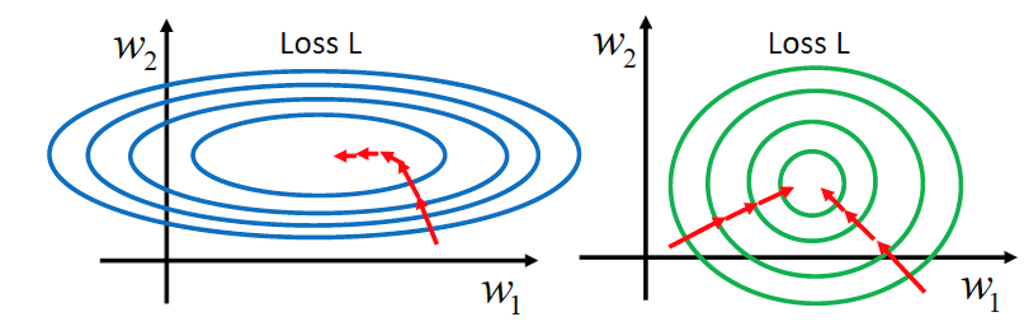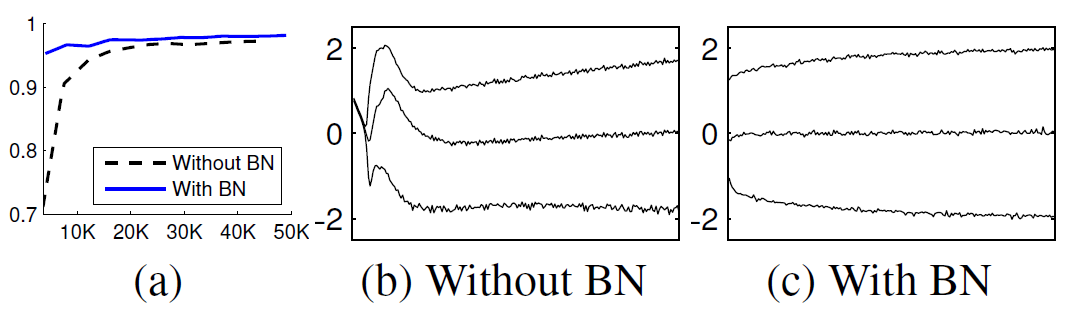# Review: Batch Normalization (Inception-v2 / BN-Inception) —The 2nd to Surpass Human-Level Performance in ILSVRC 2015 (Image Classification)

In this story, Inception-v2  by Google is reviewed. This approach introduces a very essential deep learning technique called Batch Normalization (BN). BN is used for normalizing the value distribution before going into the next layer. With BN, higher accuracy and faster training speed can be achieved.

# Intense ILSVRC Competition in 2015

The ILSVRC (ImageNet Large Scale Visual Recognition Competition) competition in 2015 has become intense!

On 6 Feb 2015, Microsoft has proposed PReLU-Net  which has 4.94% error rate which surpasses the human error rate of 5.1%.

Five days later, on 11 Feb 2015, Google proposed BN-Inception / Inception-v2  in arXiv (NOT submission to ILSVRC) which has 4.8% error rate.

Though BN did not take part in the ILSVRC competition, BN has a very good concept which has been used for many networks afterwards. And it is a 2015 ICML paper with over 6000 citations at the time I was writing this story. This is a must read item in deep learning. (Sik-Ho Tsang @ Medium)

ImageNet, is a dataset of over 15 millions labeled high-resolution images with around 22,000 categories. ILSVRC uses a subset of ImageNet of around 1000 images in each of 1000 categories. In all, there are roughly 1.2 million training images, 50,000 validation images and 100,000 testing images.

# About The Inception Versions

There are 4 versions. The first GoogLeNet must be the Inception-v1 , but there are numerous typos in Inception-v3  which lead to wrong descriptions about Inception versions. Consequently, there are many reviews in the internet mixing up between v2 and v3. Some of the reviews even think that v2 and v3 are the same with only some minor different settings.

Nevertheless, in Inception-v4 , Google has a much more clear description about the version issue:

“The Inception deep convolutional architecture was introduced as GoogLeNet in (Szegedy et al. 2015a), here named Inception-v1. Later the Inception architecture was refined in various ways, first by the introduction of batch normalization (Ioffe and Szegedy 2015) (Inception-v2). Later by additional factorization ideas in the third iteration (Szegedy et al. 2015b) which will be referred to as Inception-v3 in this report.”

Thus, when we talk about Batch Normalization (BN), we are talking about Inception-v2 or BN-Inception.

# What are covered

1. Why we need Batch Normalization (BN)?
2. Batch Normalization (BN)
3. Ablation Study
4. Comparison with the State-of-the-art Approaches

# 1. Why we need Batch Normalization (BN)?

As we should know, the input X is multiplied by weight W and added by bias b and become the output Y at the next layer after an activation function F:

Y=F(W ⋅ X+b)

Previously, F is sigmoid function which is easily saturated at 1 which easily makes the gradient become zero. As the network depth increases, this effect is amplified, and thus slow down the training speed.

ReLU is then used as F, where ReLU(x)=max(x,0), to address the saturation problem and the resulting vanishing gradients. However, careful initialization, learning rate settings are required.Without BN (Left), With BN (Right)

It is advantageous for the distribution of X to remain fixed over time because a small change will be amplified when network goes deeper.

BN can reduce the dependence of gradients on the scale of the parameters or their initial values. As a result,

1. Higher learning rate can be used.
2. The need for Dropout can be reduced.

# 2. Batch Normalization (BN)

During training, we estimate the mean μ and variance σ² of the mini-batch as shown above. And the input is normalized by subtracting the mean μ and dividing it by the standard deviation σ. (The epsilon ε is to prevent denominator from being zero) And additional learnable parameters γ and β are used for scale and shift to have a better shape and position after normalization. And output Y becomes as follows:

Y=F(BN(W ⋅ X+b))

To have a more precise mean and variance, moving average is used to calculate the mean and variance.

During testing, the mean and variance are calculated using the population.

# 3. Ablation Study

## 3.1 MNIST dataset

28×28 binary image as input, 3 FC hidden layer with 100 activations each, the last hidden layer followed by 10 activations as there are 10 digits. And the loss is cross entropy loss.(a) Accuracy: With BN (Blue), Without BN (Black dotted), (b) and (c) One typical activation from last layer

BN network is much more stable.

## 3.2 Applying BN to GoogLeNet (Inception-v1)

Besides applying BN to Inception-v1 , the main difference is that the 5×5 convolutional layers are replaced by two consecutive layers of 3×3 convolutions with up to 128 filters. This is a kind of factorization mentioned in Inception-v3 .

From the above figure, there are many settings tested:

Inception: Inception-v1 without BN
BN-Baseline: Inception with BN
BN-×5: Initial learning rate is increased by a factor of 5 to 0.0075
BN-×30: Initial learning rate is increased by a factor of 30 to 0.045
BN-×5-Sigmoid: BN-×5 but with Sigmoid

By comparing Inception and BN-Baseline, we can see that using BN can improve the training speed significantly.

By observing BN-×5 and BN-×30, we can see that the initial learning rate can be increased largely to improve the training speed even better.

And by observing BN-×5-Sigmoid, we can see that saturation problem by Sigmoid can be a kind of removed.